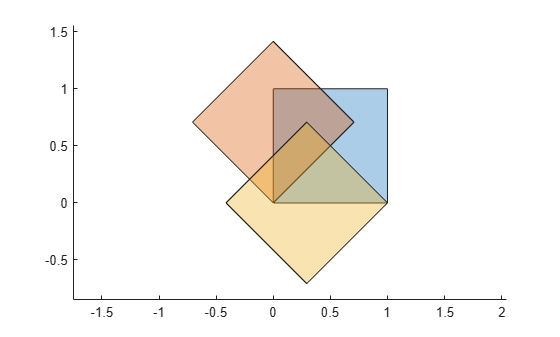# rotate

Rotate `polyshape`

## Syntax

``polyout = rotate(polyin,theta)``
``polyout = rotate(polyin,theta,refpoint)``

## Description

example

````polyout = rotate(polyin,theta)` returns a `polyshape` object created by rotating `polyin` by `theta` degrees with respect to the reference point (0,0).```

example

````polyout = rotate(polyin,theta,refpoint)` specifies a reference point to rotate with respect to.```

## Examples

collapse all

Create a square, `polyin`, and rotate it 45 degrees counterclockwise with respect to the point (0,0).

```polyin = polyshape([0 0 1 1],[1 0 0 1]); poly1 = rotate(polyin,45);```

Rotate `polyin` 45 degrees with respect to the point (1,0).

`poly2 = rotate(polyin,45,[1 0]);`

Plot all three polygons.

```plot([polyin poly1 poly2]) axis equal```## Input Arguments

collapse all

Input `polyshape`, specified as a scalar, vector, matrix, or multidimensional array.

Data Types: `polyshape`

Rotation angle, specified as a scalar number of degrees. When the input `polyshape` is an array, each element of the array is rotated by `theta` degrees. The rotation is counterclockwise when `theta` is positive, and clockwise when `theta` is negative.

Data Types: `double` | `single` | `int8` | `int16` | `int32` | `int64` | `uint8` | `uint16` | `uint32` | `uint64`

Reference point, specified as a two-element row vector containing the x-coordinate and y-coordinate. The `rotate` function rotates with respect to the reference point. When the input `polyshape` is an array, each element of the array is rotated with respect to `refpoint`.

Data Types: `double` | `single` | `int8` | `int16` | `int32` | `int64` | `uint8` | `uint16` | `uint32` | `uint64`